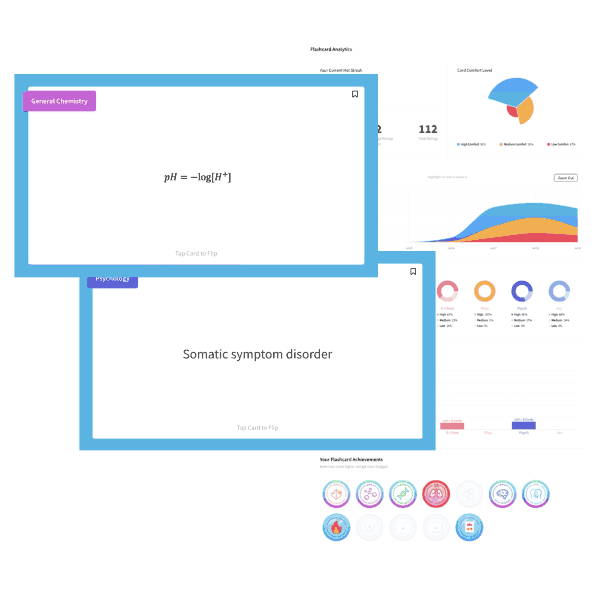# MCAT Chemistry Question — Molar Heat Capacities

The molar heat capacities (J/mole K) for zinc, copper, silver, and gold is 25.2, 24.5, 24.9, and 25.6, respectively. If 1 mole of each substance is heated until the temperature increases by 10K, which metal required the addition of the most heat?

A. Zinc

B. Copper

C. Silver

D. Gold

##### Click for Explanation

This question asks you to apply information on molar heat capacities to determine the most energetically intensive material. Since the change in temperature and the quantity of each substance is constant, the difference in molar heat capacities dictates the energy requirements for each substance.

Molar heat capacity tells you how much energy (J) is necessary to increase one mole of a substance by 1 K. Therefore, the higher the molar heat capacity, the more energy required. In the question above, gold has the highest molar heat capacity and therefore requires the greatest amount of heat. The calculations are given below.

Energy = (molar heat capacity) x (# of moles) x (change in temperature)

Zinc: (25.2 J/mole K) x (1 mole) x (10 K) = 252 J
Copper: (24.5 J/mole K) x (1 mole) x (10 K) = 245 J

Silver: (24.9 J/mole K) x (1 mole) x (10 K) = 249 J

Gold: (25.6 J/mole K) x (1 mole) x (10 K) = 256 J

Multiplying the molar heat capacity by the number of moles and the change in temperature gives you energy required. Gold requires the most heat (256 J) making answer choice D correct.

## Want more MCAT practice?

### We’ve got options for every schedule and learning style!

From the best online MCAT course created by top instructors with 524+ MCAT scores to the most representative full-length practice exams and private tutoring, we can custom tailor your MCAT prep to your goals!

Not sure which option is right for you? Schedule a free MCAT consultation with an MCAT expert using the form below. No obligation, just expert advice.

Create your Free Account to access our MCAT FlashcardsMCAT is a registered trademark of the Association of American Medical Colleges (AAMC), which is not affiliated with Blueprint.